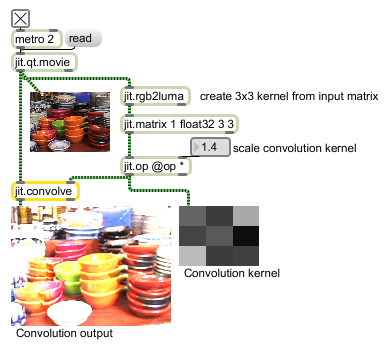# jit.convolve

Convolve two matrices

## Description

The jit.convolve object convolves a matrix with another matrix (convolution kernel). This may be used for typical image processing tasks like blurring, sharpening, and edge detection. By default, the convolution kernel is a 3 by 3 float32 matrix

## Matrix Operator

matrix inputs:2, matrix outputs:1
 Name IOProc Planelink Typelink Dimlink Plane Dim Type in2 adapt 0 0 0 1 1 float32 out n/a 1 1 1 1 1 char long float32 float64

## Attributes

Name Type g/s Description
boundmode int Boundary case handling mode (default = 0 (clip))
0 = clip: cells which lie outside the domain of the input data will clip to the nearest cell within the domain of the input data (e.g. a convolution which would need to use cell (0,-1) would instead use cell (0,0)).
1 = wrap: cells which lie outside the domain of the input data will wrap via modulus arithmetic within the domain of the input data (e.g. a convolution on a 320 by 240 matrix which would need to use cell (0,-1) would instead use cell (0,239)).
2 = ignore: cells which lie outside the domain of the input data will not be used in calculating the convolution.
origin int The origin of the convolution kernel (default = 1 1)

## Examples